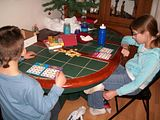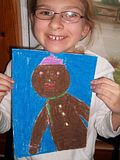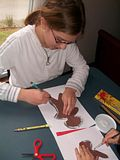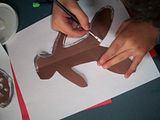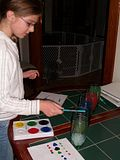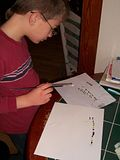## Thursday, December 16, 2010

### Unit Week Days 3 and 4

We've been doing some math games this week including Math Bingo and a game called High/Low. The students make number cards. On each turn they draw two cards and make a true math problem from the numbers. They can add, subtract, multiply or divide. If the number is more than 100--score 2 points; less than 30--score 2 points; in between 30 and 100; score 1 point. The winner after 5 rounds is the one with the highest number of points.